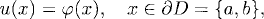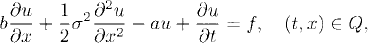# THE FEYNMAN-KAC FORMULA

The solutions to certain second-order partial differential equations can be represented as expectations of stochastic functionals. In fact, the Feynman-Kac formula establishes a link between certain partial differential equations and stochastic processes. In this chapter, we present the Feynman-Kac formula.

# 40.1 Basic Concepts and Facts

Definition 40.1 (Dirichlet Problem). Let D = (a, b) be an interval. A Dirichlet problem is an ordinary differential equation of the form

(40.1a)(40.1b)where b, σ, f, a, and ϕ are given functions, and ∂D is the boundary of of D. The function u is called a solution to the Dirichlet problem.

Definition 40.2 (Cauchy-Dirichlet Problem). Let Q = (0, T) × D, where D = (a, b) is an interval and T > 0. Let ∂pQ = ([0, T] × {a, b}) ∪ ({T} × D). A Cauchy-Dirichlet problem is a partial differential equation of the form

(40.2a)(40.2b)where b, σ, f, a, and ϕ are given functions. The function u is called a solution to the Cauchy-Dirichlet problem.

Definition 40.3 (Cauchy Problem). Let Q = (0, T) × R, where T > 0. A Cauchy problem ...

Get Measure, Probability, and Mathematical Finance: A Problem-Oriented Approach now with the O’Reilly learning platform.

O’Reilly members experience books, live events, courses curated by job role, and more from O’Reilly and nearly 200 top publishers.